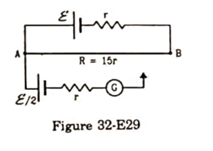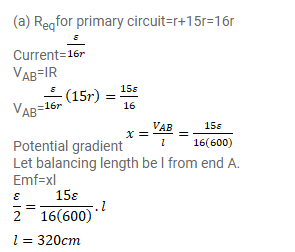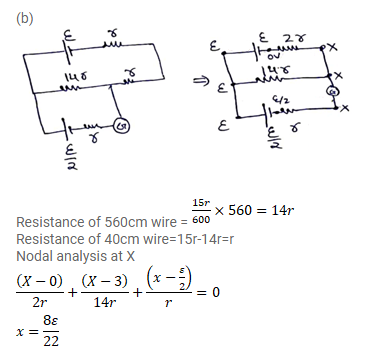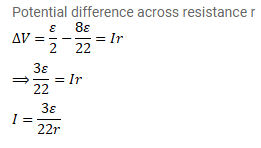# Consider the potentiometer circuit arranged as in figure (32-E29).

Question:

Consider the potentiometer circuit arranged as in figure (32-E29). The potentiometer wire is $600 \mathrm{~cm}$ long.

(a) At what distance from the point A should the jockey touch the wire to get zero deflection in the galvanometer?

(b) If the jockey touches the wire at a distance of $560 \mathrm{~cm}$ from A, what will be the current in the galvanometer?Solution: